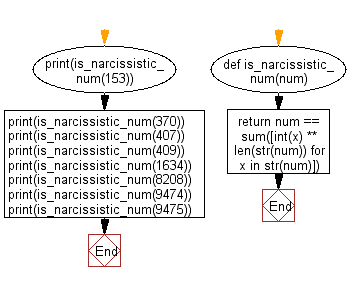﻿ Python: Check whether a given number is a narcissistic number or not - w3resource# Python: Check whether a given number is a narcissistic number or not

## Python Basic - 1: Exercise-96 with Solution

Write a Python program to check whether a given number is a narcissistic number or not.

If you are a reader of Greek mythology, then you are probably familiar with Narcissus. He was a hunter of exceptional beauty that he died because he was unable to leave a pool after falling in love with his own reflection. That's why I keep myself away from pools these days (kidding).
In mathematics, he has kins by the name of narcissistic numbers — numbers that can't get enough of themselves. In particular, they are numbers that are the sum of their digits when raised to the power of the number of digits.
For example, 371 is a narcissistic number; it has three digits, and if we cube each digits 33 + 73 + 13 the sum is 371. Other 3-digit narcissistic numbers are
153 = 13 + 53 + 33
370 = 33 + 73 + 03
407 = 43 + 03 + 73.
There are also 4-digit narcissistic numbers, some of which are 1634, 8208, 9474 since
1634 = 14+64+34+44
8208 = 84+24+04+84
9474 = 94+44+74+44
It has been proven that there are only 88 narcissistic numbers (in the decimal system) and that the largest of which is
115,132,219,018,763,992,565,095,597,973,971,522,401
has 39 digits.

Ref:: //https://bit.ly/2qNYxo2

Sample Solution:

Python Code:

``````def is_narcissistic_num(num):
return num == sum([int(x) ** len(str(num)) for x in str(num)])

print(is_narcissistic_num(153))
print(is_narcissistic_num(370))
print(is_narcissistic_num(407))
print(is_narcissistic_num(409))
print(is_narcissistic_num(1634))
print(is_narcissistic_num(8208))
print(is_narcissistic_num(9474))
print(is_narcissistic_num(9475))
``````

Sample Output:

```True
True
True
False
True
True
True
False
```

Pictorial Presentation:Flowchart:Python Code Editor:

Have another way to solve this solution? Contribute your code (and comments) through Disqus.

What is the difficulty level of this exercise?

Test your Programming skills with w3resource's quiz.

﻿

## Python: Tips of the Day

Iterating over dictionaries using 'for' loops:

I am a bit puzzled by the following code: d = {'x': 1, 'y': 2, 'z': 3} for key in d: print key, 'corresponds to', d[key] What I don't understand is the key portion. How does Python recognize ...

key is just a variable name.

```for key in d:
```

For Python 3.x:

```for key, value in d.items():
```

For Python 2.x:

```for key, value in d.iteritems():
```

To test for yourself, change the word key to poop.

In Python 3.x, iteritems() was replaced with simply items(), which returns a set-like view backed by the dict, like iteritems() but even better. This is also available in 2.7 as viewitems().

The operation items() will work for both 2 and 3, but in 2 it will return a list of the dictionary's (key, value) pairs, which will not reflect changes to the dict that happen after the items() call. If you want the 2.x behavior in 3.x, you can call list(d.items()).

Ref: https://bit.ly/37dm0Qo